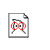# On Fitness Proportionate Selection and the Schema Theorem in the Presence of Stochastic Effects

Created by W.Langdon from gp-bibliography.bib Revision:1.7325

@TechReport{Poli00-2,
• author = "Riccardo Poli",
• title = "On Fitness Proportionate Selection and the Schema Theorem in the Presence of Stochastic Effects",
• institution = "University of Birmingham, School of Computer Science",
• number = "CSRP-00-2",
• month = feb,
• year = "2000",
• keywords = "genetic algorithms, genetic programming",
• email = "R.Poli@cs.bham.ac.uk",
• file = "/2000/CSRP-00-02.ps.gz",
• URL = "ftp://ftp.cs.bham.ac.uk/pub/tech-reports/2000/CSRP-00-02.ps.gz",
• abstract = "Holland's schema theorem has been criticised in (Fogel and Ghozeil 1997, Fogel and Ghozeil 1998, Fogel and Ghozeil 1999) for not being able to estimate correctly the expected proportion of a schema in the population when fitness proportionate selection is used in the presence of noise or other stochastic effects. This is incorrect for two reasons. Firstly, the theorem in its original form is not applicable to this case. As clarified in the paper, if the quantities involved in schema theorems are random variables, the theorems must be interpreted as conditional statements. Secondly, the conditional versions of Holland and other researchers' schema theorems are indeed very useful to model the sampling of schemata in the presence of stochasticity. In the paper I show how one can calculate the correct expected proportion of a schema in the presence of stochastic effects when selection only is present, using a conditional interpretation of Holland's schema theorem. In addition, I generalise this result (again using schema theorems) to the case in which crossover, mutation, and selection with replacement are used. This can be considered as an exact schema theorem applicable both in the presence and in the absence of stochastic effects.",
}

Genetic Programming entries for Riccardo Poli